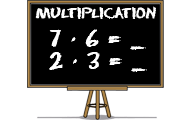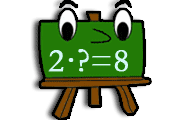# MultiplicationFiltering on knowledge field: Multiplication

Math games that will teach you about mathematics, numbers and counting. Practice the basic number perception with an abacus, or practice the four rules of arithmetic. You will learn math in a joyful way by playing these games. Here we have collected all the games on the subject Math. The games suit preschool, elementary school and all the way up to high school. The games may be sorted by popularity, target group, release date or name.
0%## Multiplication

• Numbers
• Multiplication
• Mental calculation
Learn multiplication with this math game for students. Find the product of the factors in all times tables.
0%## Multiplication memory

• Multiplication
• Tables
• Mental arithmetic
• Memory training
Practice the multiplication tables in this memory game where you should match an expression with the right product.
0%## Minecraft math

• Multiplication
• Division
• Subtraction
• Problem solving
Count with Minecraft! You will need to multiply, divide and subtract.
0%